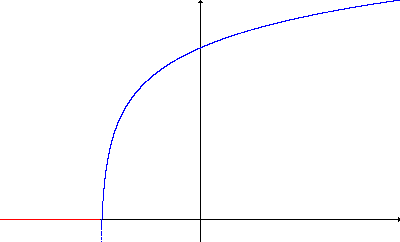# Notes on Drawing

### A)

If you specify a function that is undefined in some segments of X axis, these segments will be draw in Red, as in this example:

let's say y=log(x+50) with a range specified of -100 < x < 100

As You kow, log is undefined for negative argument, i.e. x< -50. You will get:Ý

### B)

If you choose autocentering of axes, sometimes you will NOT see axes: this is why axes are draw at x=0 and y = 0 and sometimes the origin will be skipped out:
for example if you set y= logx withÝ 100 < x < 1000 x doesn't encompass x=0 so the Y axes is not drawn; y ranges from log(100) that is 4.6 and log(100) that is 6.9 so y=0 is out and X axes will not be drawn.
Ý

### C)

If you plot on a Printer, You will be scaled accordingly, but read about resolution in "Print Command" Note